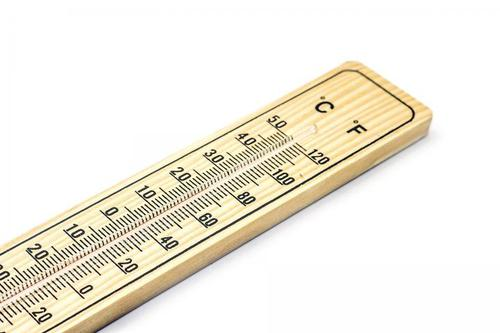### Detailed Steps for Converting Temperatures

|

Temperature is a common thing in our atmosphere and is usually measured using different temperature scales: Fahrenheit, Kelvin, and Celsius. The scales are used to measure water temperature, body temperature, room temperature, and outdoor temperature.

Before the year 1970, the standard unit of measurement of temperature was Fahrenheit. But when the popularity of the Celsius scale rose, people saw it was more reliable than the Fahrenheit scale because it was almost similar to the Kelvin scale. Nevertheless, the Fahrenheit scale is mostly used in the US and its territories. The Celsius and Kelvin scales are used widely and mostly for scientific calculation. It is imperative for people to know how to convert temperatures, especially when doing scientific research or exploring the world. Some thermometers will have both scales such as having both Celsius and Fahrenheit degrees. Here are the simple formulas on how to convert Fahrenheit to Celsius, Celsius to Fahrenheit and Celsius and Fahrenheit to Kelvin.

## How to Convert Temperatures into Different Scales

Converting temperatures into different is very simple. But, if you do not follow the formulas carefully it can get complicated. Below are some of the simplest conversion tricks.### 1. Converting Fahrenheit to Celsius

Learning how to convert Fahrenheit to Celsius is very easy. You only need to know the formula. The formulas for converting Fahrenheit to Celsius are:

• T(°C) = (T(°F) - 32) × 5/9
• T(°C) = (T(°F) - 32) / (9/5)
• T(°C) = (T(°F) - 32) / 1.8

Fahrenheit to Celsius example

Convert 90 degrees Fahrenheit to degrees Celsius: T(°C) = (90°F - 32) × 5/9 = 32.2 °C.

The T represents temperature; °C represents Celsius which is equal to Fahrenheit (°F) minus 32, times 5/9.

The above example has followed three simple steps of converting Fahrenheit to Celsius:

• By taking the Fahrenheit temperature (90°F) and subtracting 32.
• Multiplying the result in step 1 by 5.
• Divide the results in step 2 by 9.

### 2. Converting Celsius to Fahrenheit

The above example demonstrated how to convert Fahrenheit to Celsius. The next example will show how to convert Fahrenheit to Celsius.

The formulas for converting Celsius to Fahrenheit are:

• T(°F) = T(°C) × 9/5 + 32;
• T(°F) = T(°C) × 1.8 + 32

Celsius to Fahrenheit example

Convert 30 degrees Celsius to degree Fahrenheit: T(°F) = 30°C × 9/5 + 32 = 86 °F.

The T represents temperature, Fahrenheit (°F) which is equal to Celsius (°C) times 9/5 plus 32. Convert Fahrenheit to Celsius by following two steps:

• Take your Celsius temperature (30°C) and multiply it by 1.8.### 3. Converting Celsius to Kelvin

Celsius and Kelvin are the most common scientific temperature scales. It is easy to convert them because their scales have similarity in size degree.

The formula for converting Celsius to Kelvin is:

• T(K) = T(°C) + 273.15

Celsius to Kelvin example

Convert 30 degrees Celsius to Kelvin: T(K) = 30°C + 273.15 = 303.15 K.

To convert degrees Celsius to Kelvin:

Kelvin does not use the degree symbol (°). The reason for this is that Kelvin is an absolute scale while the Celsius scale follows the properties of water.

### 4. Converting Fahrenheit to Kelvin

Fahrenheit is mostly used in the USA and Kelvin is used widely for scientific calculation. It is easy to convert Fahrenheit because of a constant factor (459.67).

The formula for conversion is:

• T(K) = (T(°F) + 459.67) × 5/9

However, it has two methods.

Method 1

It involves using the above formula. For example, convert 50 degrees Fahrenheit to degrees Kelvin: T(K) = (50°F + 459.67) × 5/9 = 283.15 K.

Method 2

• Take the Fahrenheit temperature and subtract 32.
• Multiplying the result in step 1 by 5.
• Dividing the result in step 2 by 9.

### 5. Temperature Scale Conversion Shortcut

Instead of using fractions (9/5 and 5/9), use 1.8 for easier conversions. In case you want to convert Celsius into Fahrenheit, multiply the Celsius number by 1.8, and then add 32 to the product. If you want to convert Fahrenheit into Celsius, subtract 32 from the Fahrenheit number, and then divide the answer you get by 1.8. This will get your temperature in Celsius. But you can also use conversion tables. You can check a certain temperature by use of conversion tables, from Celsius to Fahrenheit and vice versa.

## Final Thoughts

Temperature conversions are straightforward; all that you need to know is the formulae of each. It can be sometimes hard to remember the formulae, but with the advancement of technology, temperature conversion is made reliable and straightforward through the apps and sites available on the Internet.

### RELATED TOPICS

By clicking into any content on this site, you agree to our privacy and cookies policy.